# Variable Runner Length Dyno Data Acquisition Unit

To acquire data and determine the most optimized intake runner length for 49ers Racing's FSAE car.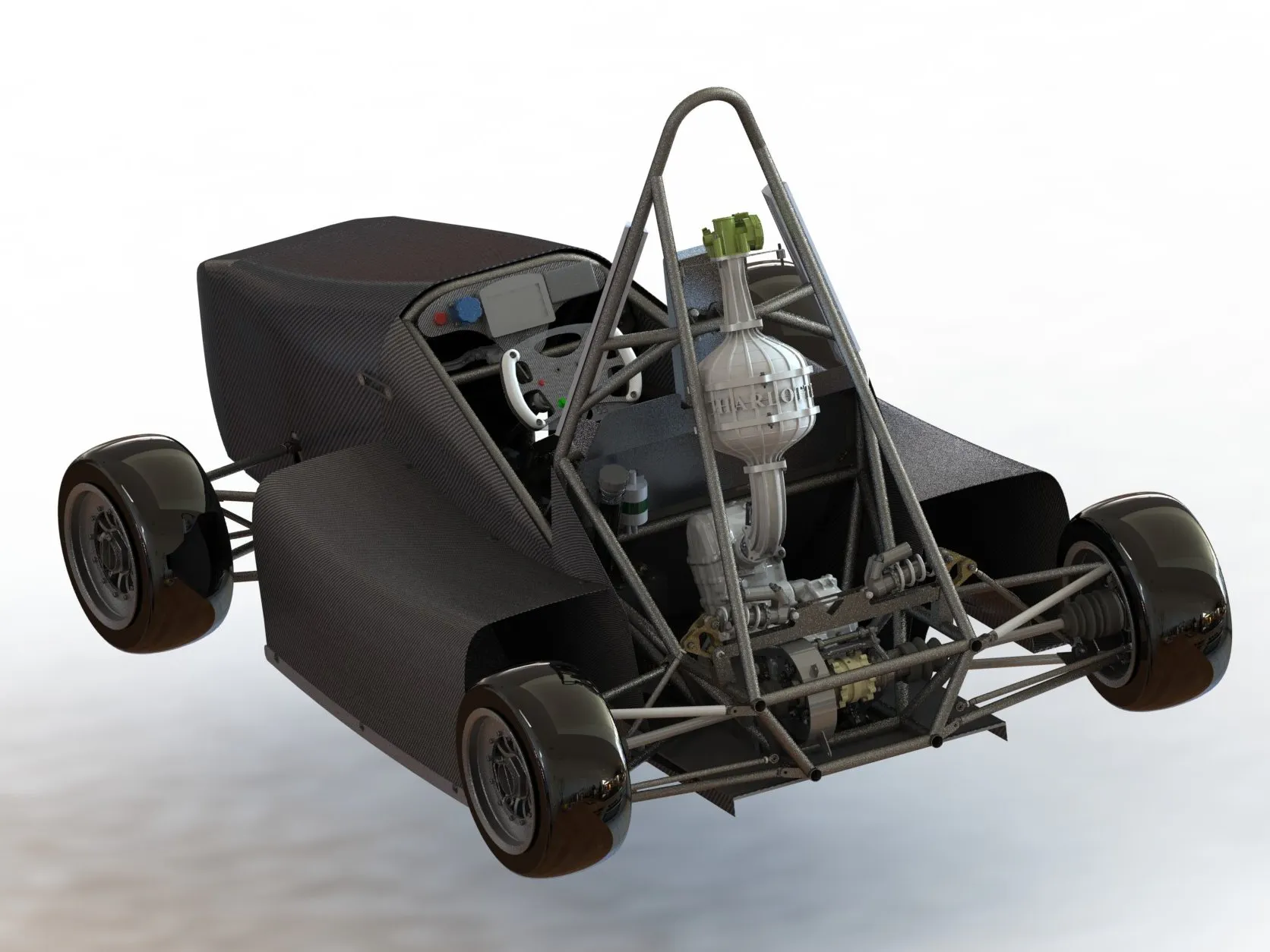## Things used in this project

### Hardware components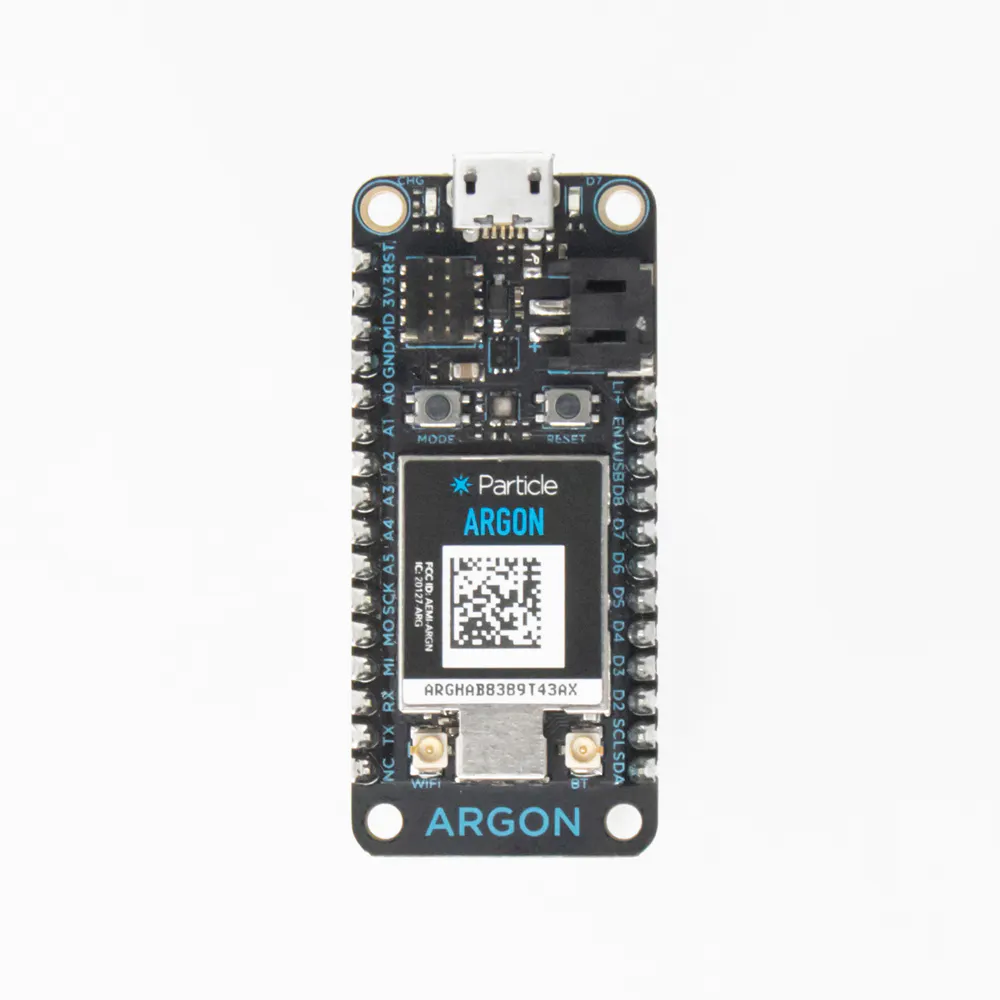Particle Argon
×2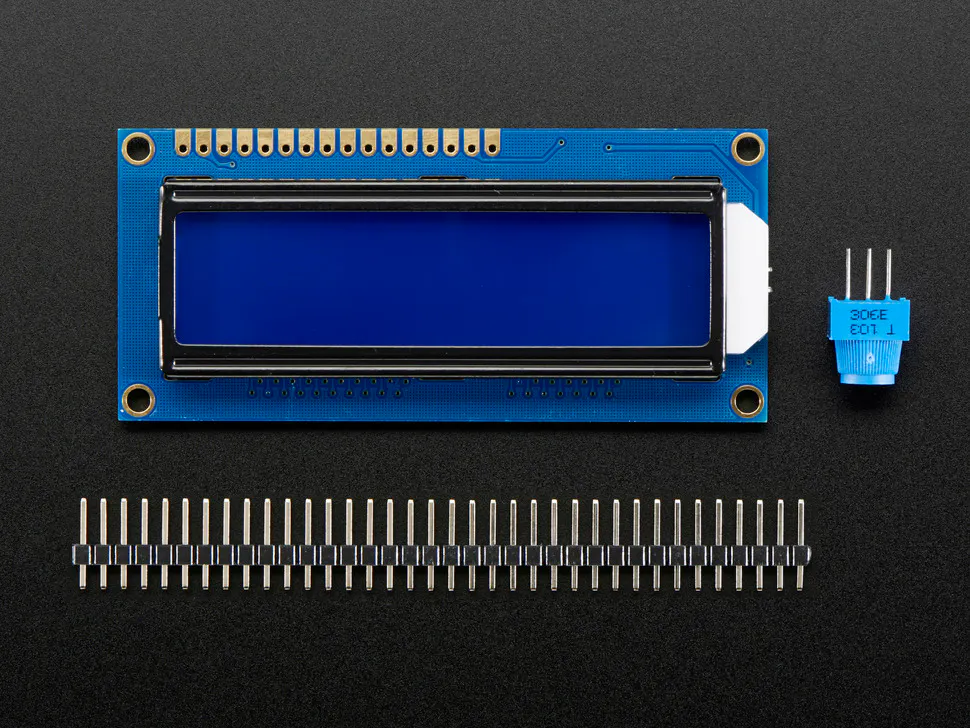Adafruit Standard LCD - 16x2 White on Blue
×1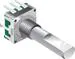Rotary Encoder with Push-Button
×1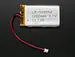Li-Ion Battery 1000mAh
×1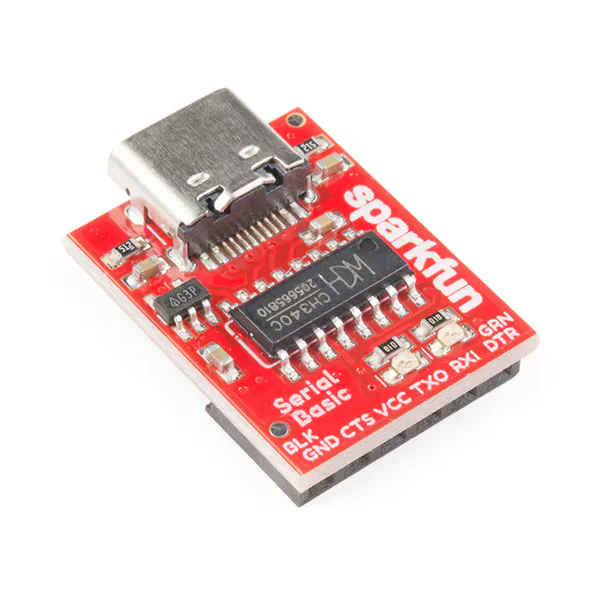SparkFun Serial Basic Breakout - CH340C and USB-C
×2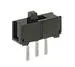Slide Switch
×1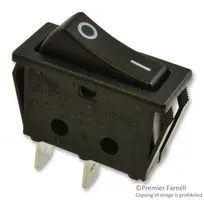Rocker Switch, Non Illuminated
×2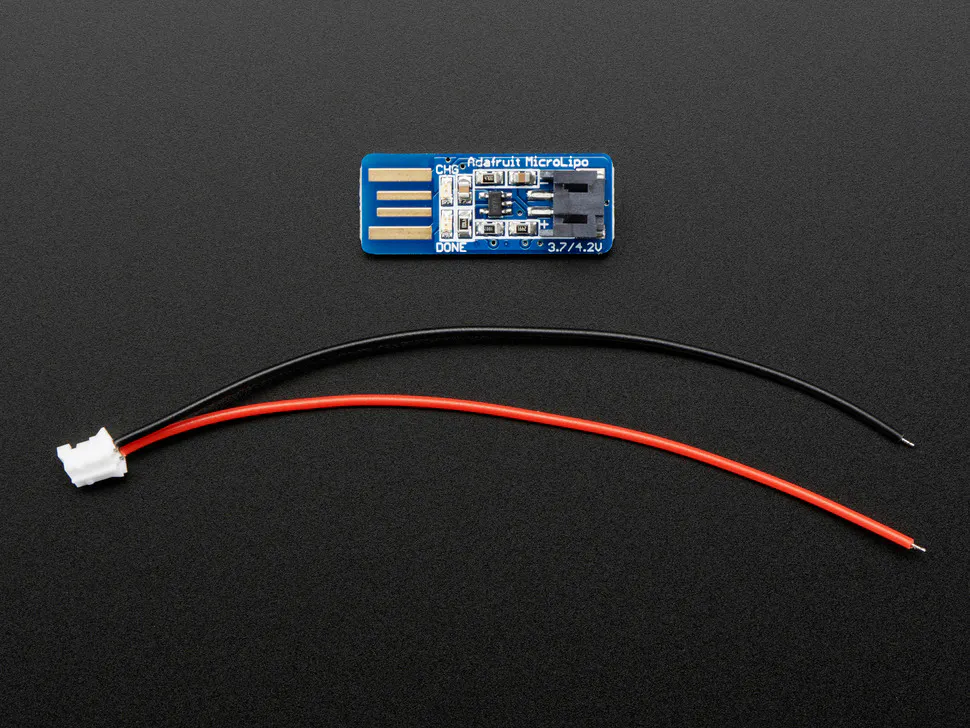Adafruit USB Li Ion Battery Charger
×1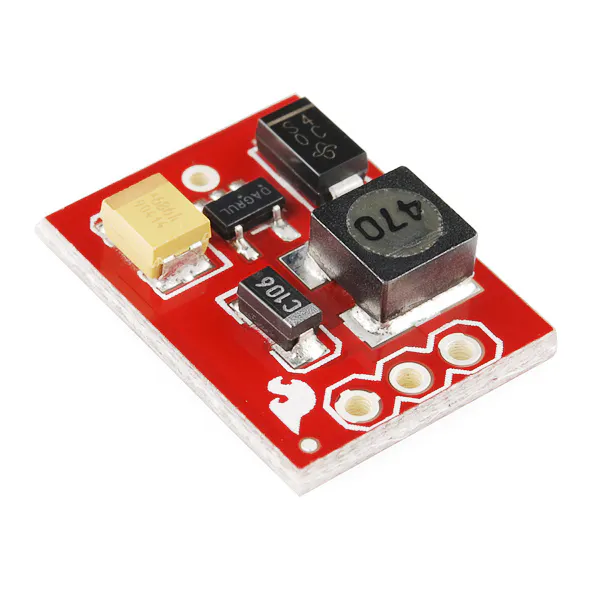SparkFun Step-Up Voltage Regulator - 3.3V
×1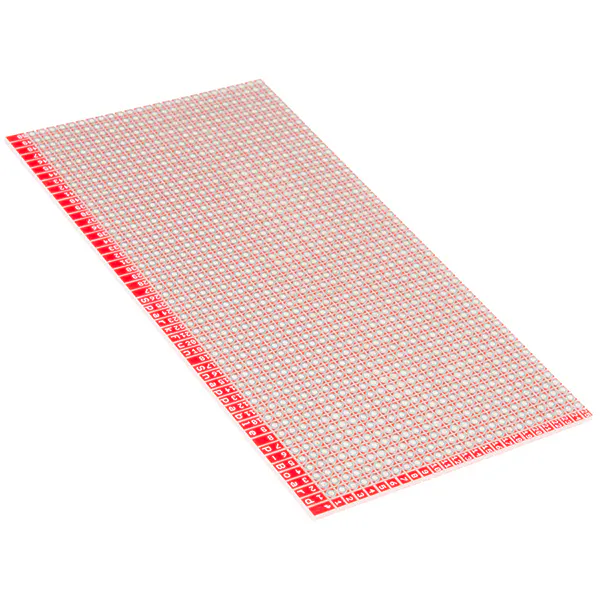SparkFun Snappable Protoboard
×2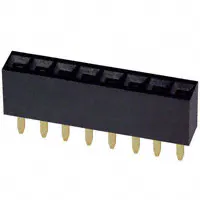Female Header 8 Position 1 Row (0.1")
×6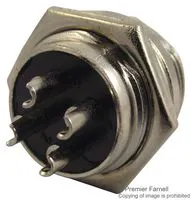Circular Connector, Panel Mount Plug
×3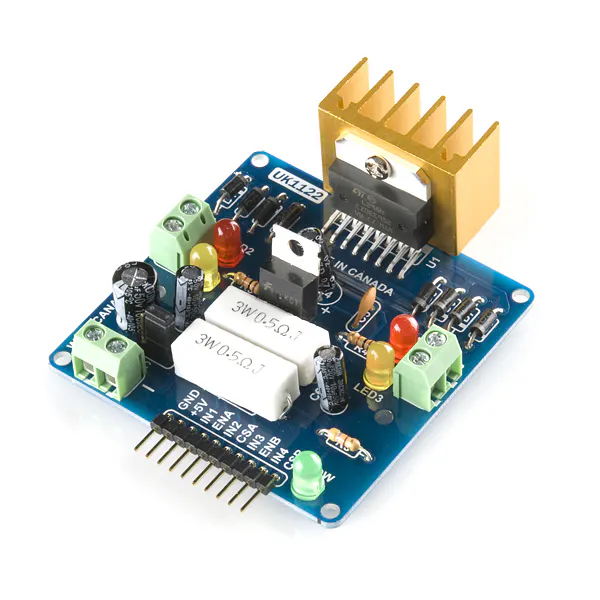SparkFun Dual H-Bridge motor drivers L298
×1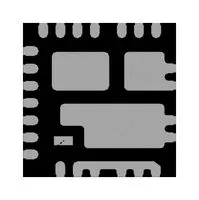DC/DC Converter, Buck Regulator
×1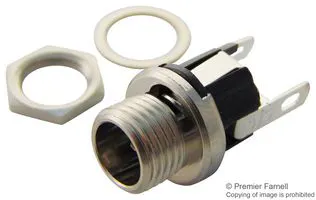DC Power Connector, Straight
×1LED (generic)
×3Jumper wires (generic)
×1
 Super Flow CFM Sensor
×1
 150mm Linear Actuator
×1

### Hand tools and fabrication machines3D Printer (generic)Soldering iron (generic)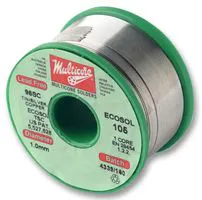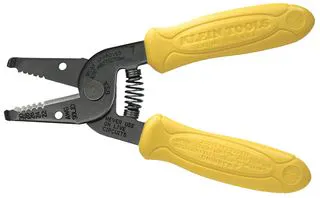Wire Stripper & Cutter, 18-10 AWG / 0.75-4mm² Capacity Wires

## Schematics

### Wireless Controller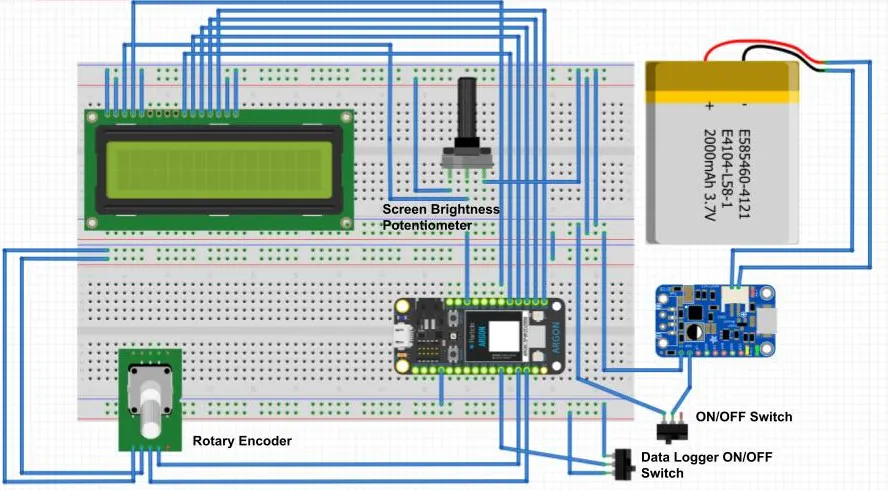### Data Acquisition Unit Control Box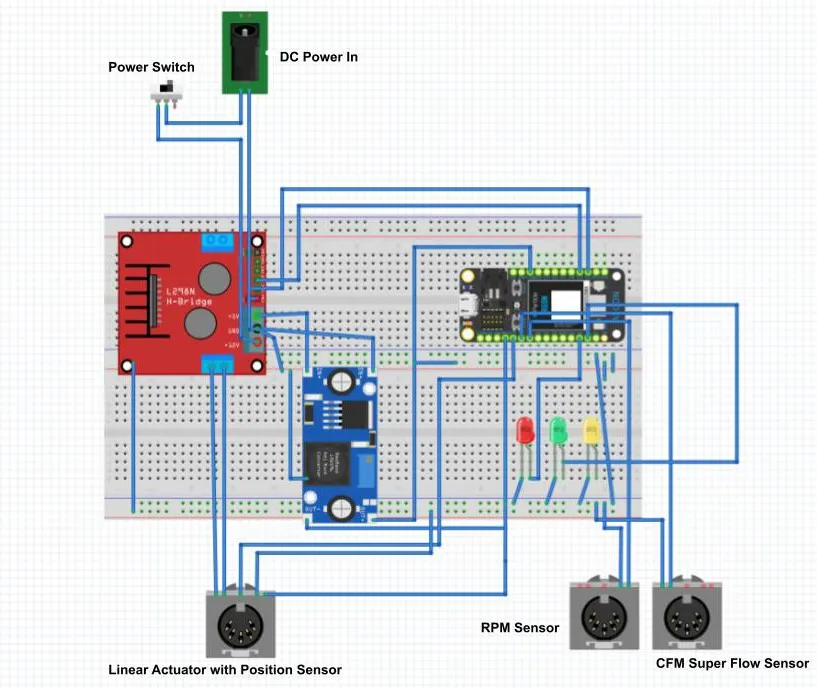## Code

### Data Acquisition Unit Control Box

C/C++
```// Pin Map
int FORWARD = D3;   //forward enable pin on motor controller
int REVERSE = D2;   //reverse enable pin on the motor controller
int ENCODER = A0;   //potentiometer connected to analog pin A1
int GREEN = D10;
int RED = D11;
int YELLOW = D9;

int IntData = 0;
int dataLit = D7;
int dataPin = D6;

//#include <time.h>
unsigned long myTime;

// Here is where we will save the temp, current, cfm vars
double cfm = 0;
double runLen = 0;
double RPM = 0;

int CFMPin = A1; //input for cfm meter
int RPMPin= A3;

void setup()
{
pinMode(FORWARD, OUTPUT);    // sets pin as output
pinMode(REVERSE, OUTPUT);    // sets pin as output
pinMode(GREEN, OUTPUT);     // sets pin as output
pinMode(RED, OUTPUT);     // sets pin as output
pinMode(YELLOW, OUTPUT);     // sets pin as output
Particle.subscribe("Data Logger", datalogger);

// Set the port speed for serial window messages
Serial.begin(9600);
Serial.println("TimeStamp, Runner Length, RPM, CFM");
}

// handles to inputs from the wireless controller
void adjustrunner(const char *event, const char *data)
{
String myData = data;
IntData =   myData.toInt();
IntData = IntData*693.42/10;
}

// handles to inputs from the wireless controller
void datalogger(const char *event, const char *data)
{
if(strcmp(data, "Door Open") == 0){
digitalWrite(dataPin, HIGH);
digitalWrite(dataLit, HIGH);
}else if (strcmp(data, "Door Closed") == 0){
digitalWrite(dataPin, LOW);
digitalWrite(dataLit, LOW);
}
}

void loop()
{
if (analogRead(ENCODER) > (IntData) + 40 ) {
digitalWrite(REVERSE, HIGH);
digitalWrite(FORWARD, LOW);
digitalWrite(GREEN, LOW);
digitalWrite(RED, HIGH);
digitalWrite(YELLOW, LOW);
}
else if (analogRead(ENCODER) < (IntData) - 40) {
digitalWrite(REVERSE, LOW);
digitalWrite(FORWARD, HIGH);
digitalWrite(GREEN, LOW);
digitalWrite(RED, LOW);
digitalWrite(YELLOW, HIGH);
}
else {
digitalWrite(REVERSE, LOW);
digitalWrite(FORWARD, LOW);
digitalWrite(GREEN, HIGH);
digitalWrite(RED, LOW);
digitalWrite(YELLOW, LOW);
}
}
Particle.publish("Runner", String(encoderPos), PUBLIC);

myTime = millis();
char dataStr = "";
char buffer;

dtostrf(myTime, 1, 0, buffer);
strcat(dataStr, buffer);
strcat(dataStr, ",");

runLen = runLen/126.5;
dtostrf(runLen, 2, 1, buffer);
strcat(dataStr, buffer);
strcat(dataStr, ",");

dtostrf(RPM, 2, 1, buffer);
strcat(dataStr, buffer);
strcat(dataStr, ",");

dtostrf(cfm, 2, 1, buffer);
strcat(dataStr, buffer);
strcat(dataStr, ",");
strcat(dataStr, 0); //terminate correctly

Serial.println(dataStr);
}

int counter = 0;
double RPm = 0;

int starttime = millis();
int endtime = starttime;
while ((endtime - starttime) <=100){
//count rotations per second based on voltage flips
counter += 1;
}
endtime = millis();
}

RPM = counter / 2*60*10;

return RPM;
}

int counter = 0;
double cfm = 0;

int starttime = millis();
int endtime = starttime;
while ((endtime - starttime) <=100){
//count rotations per second based on voltage flips
counter += 1;
}
endtime = millis();
}

counter = counter / 2*10;

// calc cfm for 4 inch turbine
cfm = 0.2378*counter + 2.9045;

return cfm;
}
```

### Wireless Controller

C/C++
```// This #include statement was automatically added by the Particle IDE.
#include <LiquidCrystal.h>
LiquidCrystal lcd(5, 4, 3, 2, 1, 0);

void doEncoderA();
void doEncoderB();

int encoderA = D10;
int encoderB = D11;
int switchPin = D13;
//int switchPin = D13;
int ledPin = D7;

volatile bool A_set = false;
volatile bool B_set = false;
volatile int encoderPos = 0;

// variables will change:
int prevPos = 0;
int value = 0;
int switchVal;
int IntData = 0;
int oldVal = LOW;

// The following line is optional, but recommended in most firmware. It
// allows your code to run before the cloud is connected. In this case,
// breathing cyan,

void setup() {
// Setup LCD
lcd.begin(16,2);
lcd.print("Runner Length:");
lcd.setCursor(0,1);
lcd.print(0.00);
lcd.setCursor(4,1);
lcd.print("in");
pinMode(encoderA, INPUT_PULLUP);
pinMode(encoderB, INPUT_PULLUP);
pinMode(ledPin, OUTPUT);
pinMode(switchPin, INPUT);
attachInterrupt(encoderA, doEncoderA, CHANGE);
attachInterrupt(encoderB, doEncoderB, CHANGE);
Serial.begin(9600);
Particle.subscribe("Runner", printdata);
}

void loop() {
if (prevPos != encoderPos) {
prevPos = encoderPos;
lcd.setCursor(0,1);
lcd.print(encoderPos*0.1);
Particle.publish("Runner_Length", String(encoderPos), PUBLIC);
}

if ((switchVal == HIGH)&& (oldVal == LOW)){
digitalWrite(ledPin, HIGH);
Particle.publish("Data Logger", "Door Open", PUBLIC);
oldVal = HIGH;
}
else if ((switchVal == LOW)&& (oldVal == HIGH)){
digitalWrite(ledPin, LOW);
Particle.publish("Data Logger", "Door Closed", PUBLIC);
oldVal = LOW;
}
}

// handles to inputs from the wireless controller
void print data(const char *event, const char *data)
{
lcd.setCursor(8,1);
lcd.print(data);
lcd.setCursor(12,1);
lcd.print("in");
}

//----------------------------------------------------------------
void doEncoderA(){
if( digitalRead(encoderA) != A_set ) {  // debounce once more
A_set = !A_set;
if ( A_set && !B_set )
if (encoderPos < (150/25.4/0.1)-0.1)
{
encoderPos += 1;
}
}
}

// Interrupt on B changing state, same as A above
void doEncoderB(){
if( digitalRead(encoderB) != B_set ) {
B_set = !B_set;
if( B_set && !A_set )
if (encoderPos > 0)
{
encoderPos -= 1;
}
}
}
```

### Analysis Code

MATLAB
```%% This programs RPM versus Volumetric Efficiency to determine optimal Runner Length

%By: Joseph Cranfill
% Date: 11/08/2022

clear
clc
close all

%% Inputs
% log file from arduino

% Manifold absolute pressure
MAP = 101.325; % KPa

% Intake air temperature of Rankine
IAT  = 22; % deg C

% Engine displacment in CC
DIS = 450; % CC

% Dyno Air Density
AirDen = 0.072; % lb/ft^3

% Turn on or off best fit lines
fit = false;

% Polynomial Degree
deg = 12;

%% Constants
% Linear Actuator
minPos = 0; %in
maxPos = 5.9; %in
resolution = 0.1; %in

%% --------------------- DO NOT EDIT BELOW THIS LINE ---------------------
% Pulls CFM, RMP and Runner Length from the data log
data = table2array(data);
MAFmeasured = data(:,4);
RPM = data(:,3);

%% Conversions
% CC to cubic inches
DIS = DIS/16.387;

% Celsius to Rankine
IAT = IAT * (9/5) + 491.67;

% KPa to PSI
MAP = MAP / 6.895;

% Converts CFM to lb/min
MAFmeasured = MAFmeasured*AirDen;

%% Calculations
% Calculates the theroretical Mass AirFlow in lb/min
MAFtheoretical = ((DIS*(RPM./2))/12^3)*(2.7*(MAP/IAT));

% calculates volumetric efficiency
VE=MAFmeasured./MAFtheoretical*100;

%% Compile data into one matrix
% Timestamp, runner length, rpm, MAF Measured, MAF Theoretical, Volumetric
% Efficiency
data = cat(2,data,MAFmeasured, MAFtheoretical, VE);
% clears matrixes used for calculations
clear RPM
clear MAFtheoretical
clear MAFmeasured
clear VE
data(:,1) = [];
data(:,3) = [];
data(:,3) = [];
data(:,3) = [];

% Seperates all the data based on runner length
for i = (minPos +1):((maxPos/resolution)+1)
Length{i}=[data(data(:,1) == (i-1)*resolution,2),data(data(:,1)== (i-1)*resolution,3),data(data(:,1)== (i-1)*resolution,1)];
end

%% Graph
% Calculates the max RPM from the collected data
maxRPM = 0;
for row = 1:size(data,1)
if abs(data(row,2)) > abs(maxRPM)
maxRPM = abs(data(row,2));
end
end

x = 0:1:maxRPM;

for j = (minPos +1):((maxPos/resolution)+1)
str=sprintf('L = %2.2f in',((j-1)*resolution));
if fit == 1
matrix = isempty(Length{j});
plot(Length{j}(:,1),Length{j}(:,2),'.','HandleVisibility','off')
hold on
if matrix == 0
p{j} = polyfit(Length{j}(:,1),Length{j}(:,2),deg);
y{j} = polyval(p{j},x);
plot(x,y{j},'DisplayName',str)
hold on
end
else
plot(Length{j}(:,1),Length{j}(:,2),'.','DisplayName',str)
hold on
end
end

grid on
set(gca, 'fontsize', 14)
xlabel ('Engine Speed(RPM)')
ylabel ('Volumetric Efficiency(%)')
title ('Volumetric Efficiency with Varying Runner Lengths')
legend
legend('Location','eastoutside')
```

## Credits

### Joseph Cranfill

1 project • 2 followers

### Isaac Polson

1 project • 1 follower